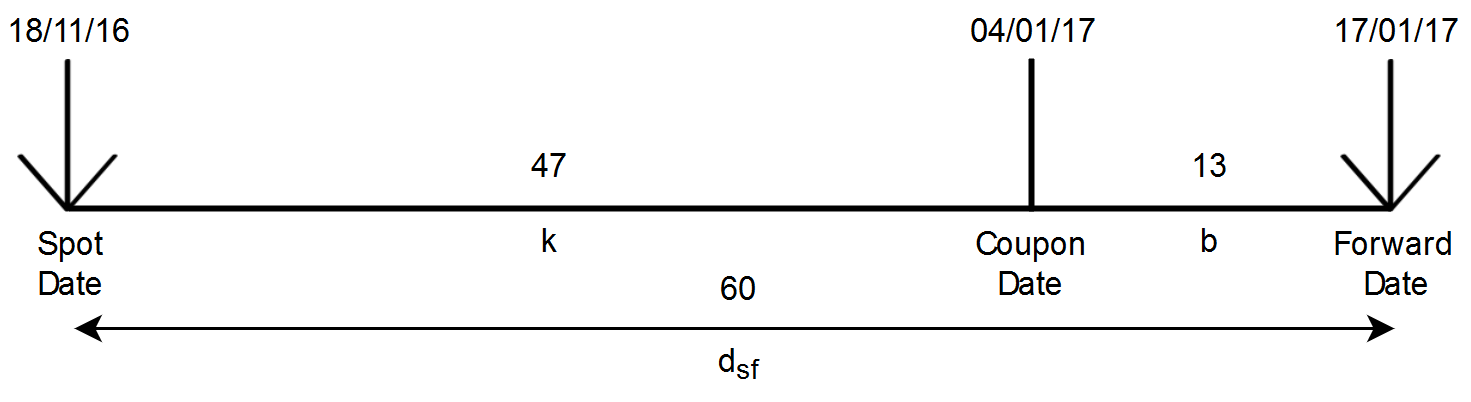# Forward Price Analysis of Coupon BondsTwo-way bond prices advertised by dealers on electronic trading platforms are valid for immediate delivery. These prices are called spot prices. A client that wishes the security to be delivered at a particular date in the future will implicitly enter in a forward contract with the dealer. The forward price refers to the price of that contract. The term forward date refers to the date of that future purchase or sale. By convention, the forward price is quoted as a flat price. The invoice price of the transaction equals the forward price plus accrued interest at the forward date.

The concept behind the forward price calculation is to equalize income and expenses for the following strategy:

1. Borrow the money at repo rate R
2. Buy the bond at spot date
3. At forward date sell the bond for forward price Pf

In other words:

Full price at spot date + financing cost from spot date to forward date = forward price + coupon payments from spot date to forward date + reinvestment of coupon payments until forward date + accrued interest at forward date

Three methods are used in practice to calculate the forward price. They vary in the calculation of financing cost on borrowed money and on the reinvestment of coupon payments received between the spot date and the forward date. We’ll consider the simple use case of one coupon payment between the spot date and the forward date for the three methods.

Name Value Description
Pf   Forward price per 100 currency units of face value
Ps 109.502045 Spot price per 100 currency units of face value
AIs 2.8326502732 Accrued Interest at Spot date per 100 currency units of face value
R 0.015 Repo rate expressed as decimal
dsf 60 Actual number of days between the Spot date and the Forward date
AIf 0.1157534247 Accrued Interest at Forward date per 100 currency units of face value
C 3.25 Coupon payment per 100 currency units of face value
k 47 Actual number of days between the Spot date and the Coupon date
b 13 Actual number of days between the Coupon date and the Forward date

The below diagram illustrates the key dates:## Proceeds Method

The financing cost on the invoice price is calculated using simple interest from the spot date to the forward date.

Coupon is reinvested with simple interest from the coupon date to the forward date.

$(P_{s}+AI_{s}) \left(1+\frac{R\cdot d_{sf}}{360}\right) = P_{f}+AI_{f}+C\left(1+\frac{R\cdot b}{360}\right)$

Or, rearranging terms,

$P_{f} = (P_{s}+AI_{s}) \left(1+\frac{R\cdot d_{sf}}{360}\right)-AI_{f}- C\left(1+\frac{R\cdot b}{360}\right)$

Substituting the values gives:

$P_{f} = (109.502045+2.8326502732) \left(1+\frac{0.015\cdot 60}{360}\right)-0.1157534247 -3.25\left(1+\frac{0.015\cdot 13}{360}\right) =\textbf{109.2480182}$

## CD Method

The financing cost on the invoice price is calculated by combining simple interest from the spot date to the coupon date with simple interest from the coupon date to the forward date.

Coupon is reinvested with simple interest from the coupon date to the forward date.

$P_{f} = (P_{s}+AI_{s}) \left(1+\frac{R\cdot k}{360}\right)\left(1+\frac{R\cdot b}{360}\right)-AI_{f}- C\left(1+\frac{R\cdot b}{360}\right)$

Substituting the values gives:

$P_{f} = (109.502045+2.8326502732) \left(1+\frac{0.015\cdot 47}{360}\right)\left(1+\frac{0.015\cdot 13}{360}\right)-0.1157534247 -3.25\left(1+\frac{0.015\cdot 13}{360}\right) =\textbf{109.2481373}$

## Scientific Method

The financing cost on the invoice price is calculated by compounding interest from the spot date to the forward date.

Coupon reinvestment is calculated by compounding interest from the coupon date to the forward date.

$P_{f} = (P_{s}+AI_{s}) \left(1+R\right)^{\frac{d_{sf}}{360}}-AI_{f}- C\left(1+R\right)^{\frac{b}{360}}$

Substituting the values gives:

$P_{f} = (109.502045+2.8326502732) \left(1+0.015\right)^{\frac{60}{360}}-0.1157534247- 3.25\left(1+0.015\right)^{\frac{13}{360}}=\textbf{109.2462915}$

FD = Ps - Pf

IPs = Ps + AIs

IPf = Pf + AIf